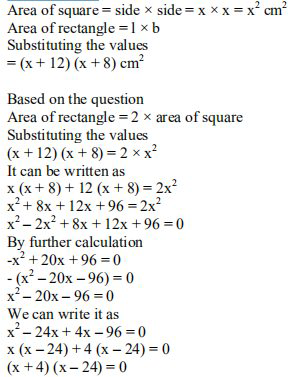Ml aggarwal solutionsOur top 5% students will be awarded a special scholarship to Lido.
Set your child up for success with Lido, book a class today!

A rectangle has twice the area of a square. The length of the rectangle is 12 cm greater and the width is

8 cm greater than 2 side of a square. Find the perimeter of the square.Looking to do well in your science exam ? Learn from an expert tutor. Book a free class!

Consider

Side of a square = x cm

Length of rectangle = (x + 12) cm

Breadth of rectangle = (x + 8) cm

We know thatHere

x + 4 = 0 or x – 24 = 0

x = -4 or x = 24 cm

Side of square = 24 cm

The perimeter of square = 4 × side

Substituting the values

= 4 × 24

= 96 cm

Our top 5% students will be awarded a special scholarship to Lido.Lido

Courses

Teachers

Book a Demo with us

Syllabus

Science
English
Coding

Terms & Policies

NCERT Question Bank

Maths
Science

Selina Question Bank

Maths
Physics
Biology

Allied Question Bank

Chemistry